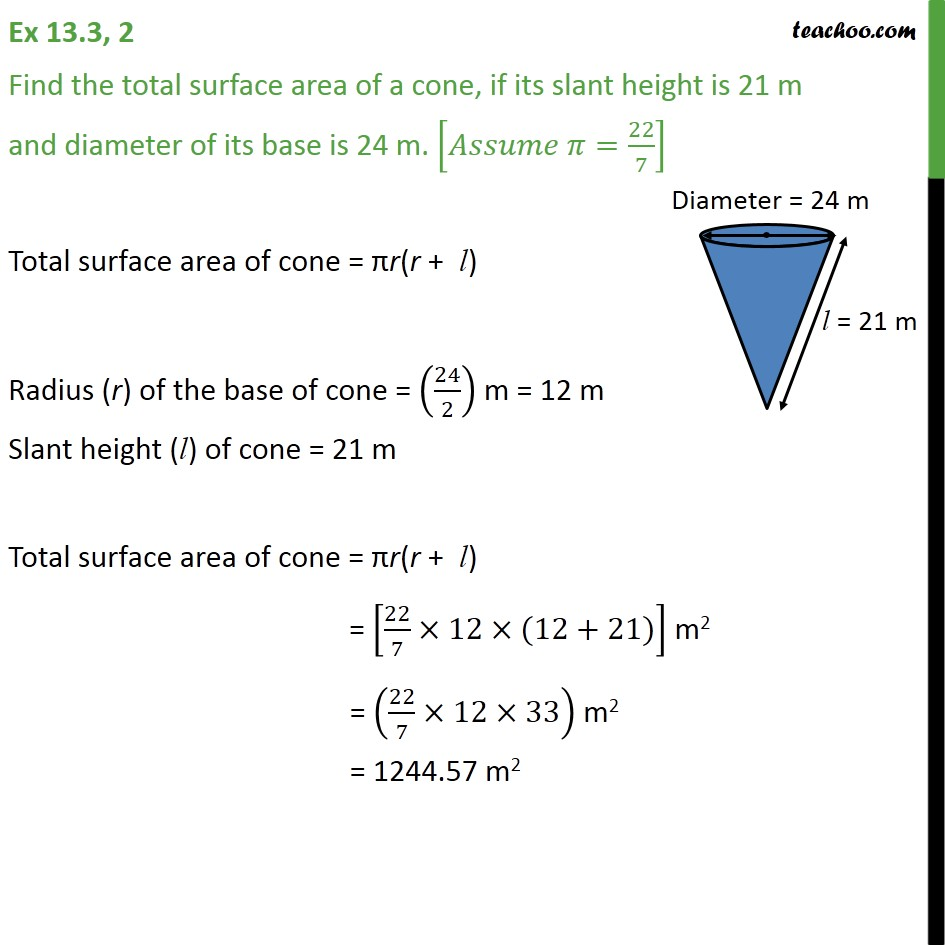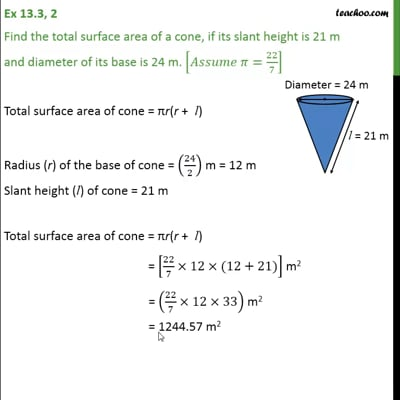Ex 13.3

Chapter 13 Class 9 Surface Areas and Volumes
Serial order wiseThis video is only available for Teachoo black users

Solve all your doubts with Teachoo Black (new monthly pack available now!)

### Transcript

Ex 13.3, 2 Find the total surface area of a cone, if its slant height is 21 m and diameter of its base is 24 m. [𝐴𝑠𝑠𝑢𝑚𝑒 𝜋=22/7] Total surface area of cone = πr(r + l) Radius (r) of the base of cone = (24/2) m = 12 m Slant height (l) of cone = 21 m Total surface area of cone = πr(r + l) = [22/7×12×(12+21)] m2 = (22/7×12×33) m2 = 1244.57 m2